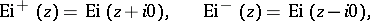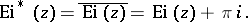# Integral exponential function

Jump to: navigation, search

The special function defined for real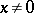by the equation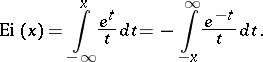The graph of the integral exponential function is illustrated in Fig..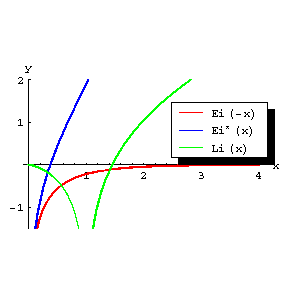Figure: i051440a

Graphs of the functions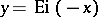,and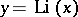.

For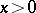, the functionhas an infinite discontinuity at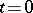, and the integral exponential function is understood in the sense of the principal value of this integral: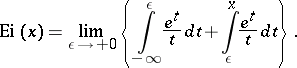The integral exponential function can be represented by the series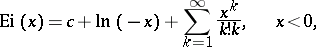(1)

and(2)

whereis the Euler constant.

There is an asymptotic representation: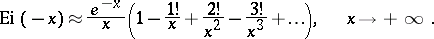As a function of the complex variable, the integral exponential function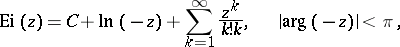is a single-valued analytic function in the-plane slit along the positive real semi-axis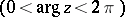; here the value of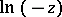is chosen such that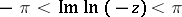. The behaviour ofclose to the slit is described by the limiting relations: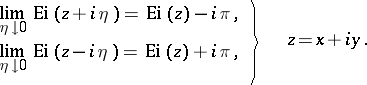The asymptotic representation in the region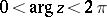is:The integral exponential function is related to the integral logarithm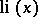by the formulas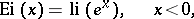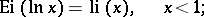and to the integral sine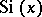and the integral cosine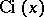by the formulas: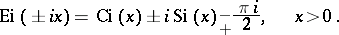The differentiation formula is: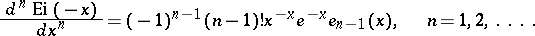The following notations are sometimes used: